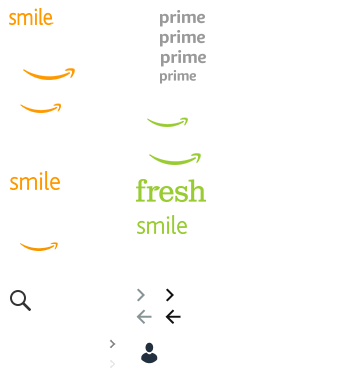# Currency Converter Supported Currencies

The Amazon Currency Converter is available when you use either an eligible credit or debit card denominated in a supported currency.

The Amazon Currency Converter supports these currencies:

Currency American Express MasterCard Visa
Argentine Peso (ARS) x x
Armenian Dram (AMD) x x x
Aruban Florin (AWG) x x x
Australian Dollar (AUD) x x x
Azerbaijanian Manat (AZN) x x x
Bahamian Dollar (BSD) x x x
Barbadian Dollar (BBD) x x x
Belize Dollar (BZD) x x x
Bermudan Dollar (BMD) x x x
Boliviano (BOB) x x
Brazilian Real (BRL) x x
Brunei Dollar (BND) x x x
Bulgarian Lev (BGN) x x x

x x
Cambodian Riel (KHR) x x x
Cayman Islands Dollar (KYD) x x x
CFP Franc (XPF) x x
CFA Franc BCEAO (XOF) x x
CFA Franc BEAC (XAF) x x
Chilean Peso (CLP) x x
Chinese Yuan (CNY)

x x
Colombian Peso (COP) x x
Cordoba Oro (NIO) x x
Costa Rica Colon (CRC) x x
Czech Koruna (CZK) x x x
Danish Krone (DKK) x x x
Dominican Republic Peso (DOP) x x x
East Caribbean Dollar (XCD) x x x
Egyptian Pound (EGP) x x x
Euro (EUR) x x x
Georgian Lari (GEL) x x x
Ghana Cedi (GHS) x x x
Guatemalan Quetzal (GTQ) x x
Guyana Dollar (GYD) x x x
Hong Kong Dollar (HKD) x x x
Hungarian Forint (HUF) x x x
Indian Rupee (INR) x x x
Indonesian Rupiah (IDR) x x x
Israeli Shekel (ILS) x x x
Jamaican Dollar (JMD) x x x
Japanese Yen (JPY) x x x
Kazakhstani Tenge (KZT) x x x
Kenyan Shilling (KES) x x x
Lebanese Pound (LBP) x x x
Lempira (HNL) x x
Macau Pataca (MOP) x x x
Malaysian Ringgit (MYR) x x x
Mauritius Rupee (MUR) x x x
Mexican Peso (MXN) x x x
Moroccan Dirham (MAD) x x x
Namibia Dollar (NAD) x x x
New Zealand Dollar (NZD) x x x
Nigerian Naira (NGN) x x x
Norwegian Krone (NOK) x x x
Pakistan Rupee (PKR) x x x
Panamanian Balboa (PAB) x x
Paraguay Guarani (PYG) x x
Peruvian Nuevo Sol (PEN) x x
Polish Zloty (PLN) x x x
Pound Sterling (GBP) x x x
Qatari Riyal (QAR) x x x
Romanian Leu (RON) x x x
Rufiyaa (MVR) x x x
Russian Ruble (RUB) x x x
Saudi Arabian Riyal (SAR) x x x
Singapore Dollar (SGD) x x x
South African Rand (ZAR) x x x
South Korean Won (KRW) x x
Sri Lanka Rupee (LKR) x x x
Swedish Krona (SEK) x x x
Swiss Franc (CHF) x x x
Taiwan New Dollar (TWD) x x x
Tanzanian Shilling (TZS) x x x
Thai Baht (THB) x x x
The Philippine Peso (PHP) x x x
Trinidad and Tobago Dollar (TTD) x x x
Tugrik (MNT) x x x
Turkish Lira (TRY) x x x
United Arab Emirates Dirham (AED) x x x
Uruguayan Peso (UYU) x x
Uzbekistan Sum (UZS) x x x
Vietnamese Dong (VND) x x